# Conic Sections and Constructions

Mathematics curricula are ever changing to meet changing needs. In order to make room for new subjects it becomes necessary to downsize others. One subject that has received the short shrift is the study of conic sections. I have no strong opinions about the judgment of this change, but I do regret the loss. This is such a fascinating study, and it appeals to so many levels.

My original intention for this article was to show some constructions related to conics. The constructions are still here, but I decided that they should be supported by an explanation of some of the conic properties that were used. As it turned out, the conics defied all of my attempts at a concise summary, so I have the more expanded version below. As in the Greek tradition, my interest lies mainly in the geometric properties, so analytic models are omitted, at least for now.

Conic Constructions – This is a collection of constructions with conics. Some of them define a conic section locus given certain parameters. Others have the section as a given object from which certain points and lines are constructed.

The Conics of Apollonius – Near the year 200 BC, Apollonius of Perga produced an eight-volume treatise on conics. Most of it still survives. Here are dynamic Geometer’s Sketchpad constructions illustrating each proposition of that work.

#### Web Sketchpad (the red points)

Watch for the sketches that have red points. Those are dynamic geometry images. The developers of The Geometer’s Sketchpad have been working to make Sketchpad documents that can be viewed on a web browser. Several of them are among the images below. The images can be manipulated by dragging the red points.

#### Surface Intersections

Conic sections are planar curves, but they derive their name from a spatial model. The curve can be the intersection of a cone and a plane. There have been different variations on that model following different notions of what a cone is.

When Archimedes mentioned a cone, he was usually (not always) referring to a right circular cone. Begin with a circle. A line through the center and normal to the plane of the circle is the axis. Somewhere on the axis is the vertex. Generators are the line segments connecting each point of the circle with the vertex. Together the generators form the lateral surface of the cone. The base may or may not be considered part of the surface.

Now, still using the Archimedes model, a plane is drawn normal to one of the generators. The intersection of the plane and the surface of the cone is a conic section. The class of the conic section depends on the vertex angle.

 ellipseacute vertex angle parabolaright vertex angle hyperbolaobtuse vertex angle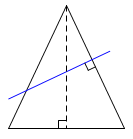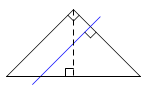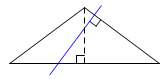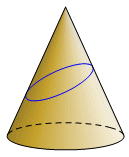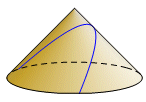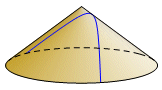This particular model cannot be used with all conic sections. For example, a circle cannot be modeled this way. Apollonius of Perga preferred an oblique cone, and the intersecting plane did not have to be perpendicular to any of the generators. Eventually, it became common to use a cone with two nappes. This fits particularly well with a hyperbola, which can be represented as a curve with two separate parts. A cylinder might even be used in place of the cone.
 circleoblique cone cut by planeparallel to base hyperbolaoblique cone with two nappes ellipsecylinder cut by oblique plane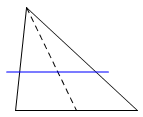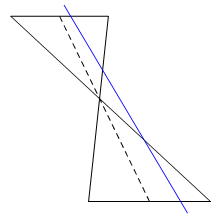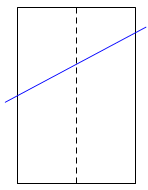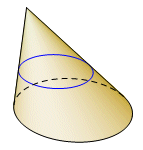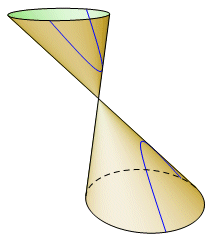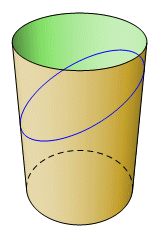0 < PF : PQ < 1 ellipse PF : PQ = 1 parabola 1 < PF : PQ hyperbola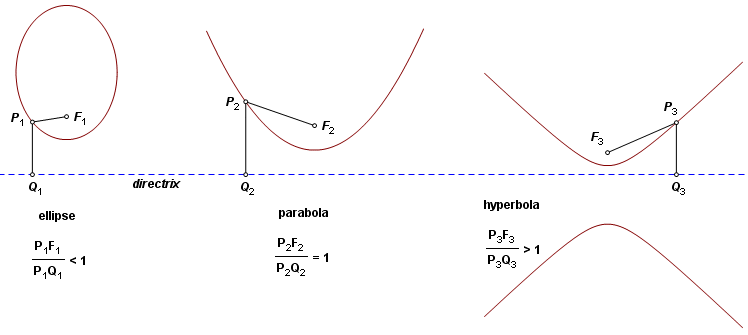Certain degenerate cases can be defined with this model by letting the focus lie on the directrix or by making the eccentricity zero. The circle, however, will not fit well even as a limiting case. We might try moving the directrix to a line at infinity and put the focus at the circle center. Every point on the circle would return a constant ratio of zero. Unfortunately though, so would every other point in the plane. A circle cannot be defined with this model. Consider an ellipse or hyperbola with vertices V1 and V2. Let F be a focus, and let Q be the point where the focal axis intersects the directrix corresponding to F. The eccentricity, e, may be expressed by either of these ratios:This proportion also means that the ellipse (or hyperbola) divides the line segment FQ harmonically in ratio e. Stated another way, points F and Q are inversion images of each other in the circle having diameter V1V2.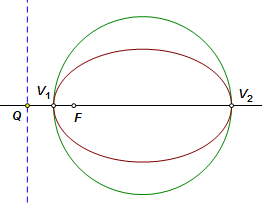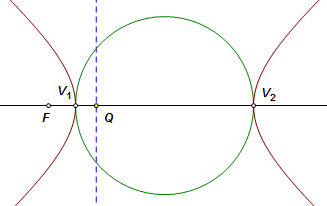Let F1 and F2 be the foci of an ellipse, and let P be a moving point on the curve. The sum of the distances PF1 and PF2 is constant. The internal bisector of ∠F1PF2 is normal to the curve. The external bisector of ∠F1PF2 is tangent to the curve. A circle is a limiting case of an ellipse where the foci are coincident. In that case the single focus is the center of the circle.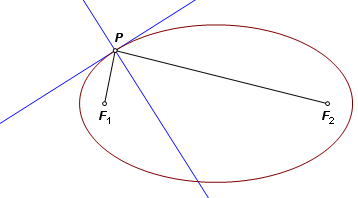Let F1 and F2 be the foci of a hyperbola, and let P be a moving point on the curve. The difference of the distances PF1 and PF2 is constant. The internal bisector of ∠F1PF2 is tangent to the curve. The external bisector of ∠F1PF2 is normal to the curve.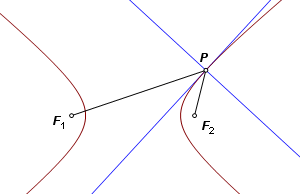The parabola is again somewhat troublesome, this time because it has no second focus. Still it may be viewed as a limiting case of either the ellipse or the hyperbola. Start with either of these curves, and send the second focus to a point at infinity in the direction of the axis. Now it is a parabola. Construct two lines through P, one through the focus and the other parallel to the axis. Bisect their angle of intersection. One bisector is normal to the curve. The other is tangent.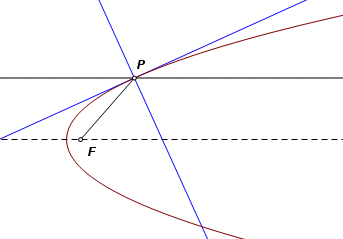#### Transformation of Conics

The isometric transformations include translation, reflection, rotation, and any combination of these. By definition, these transformations preserve distance, and because of that, they also preserve angles. Under an isometric transformation, the image of any figure is congruent to the pre‑image. Dilation is not isometric. It does not preserve distances, but it does preserve ratios of distances, hence angles. Under dilation, images are similar to their pre‑images. Under any of the above transformations, a conic can only be transformed to another conic.

The really interesting property comes under two other transformations, one-dimensional scaling and shear. These transformations do not preserve distances, ratios, or angles. Images generally are not similar to pre‑images. However, although ratios in general are not preserved, ratios of distances measured in parallel directions are preserved. Consider what this means for the ratios discussed in the abscissa/ordinate section. After transformation, they must still be constant. A conic section may change shape considerably under a scaling or shear transformation, but its image will still be a conic of the same class.

In the image below, an ellipse, a parabola, and a hyperbola are subjected to scaling and shear. Their common axis is the invariant line.

Drag the red points to see the relationships.

The foci and directrices are not shown here, because they do not retain their properties under the transformation.

When the asymptotes of the hyperbola are subjected to the same transformation, they remain asymptotic. Upon scaling, the invariant axis remains an axis, but under the shear it becomes an oblique diameter.

There is an interesting exception to the non‑similarity rule. The image of the parabola is similar to the pre‑image. In fact, all parabolas are similar.